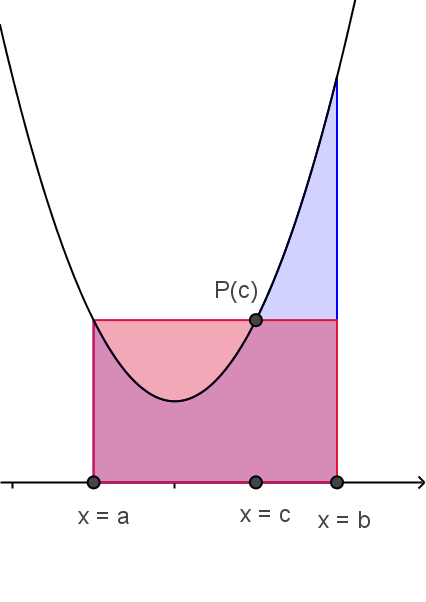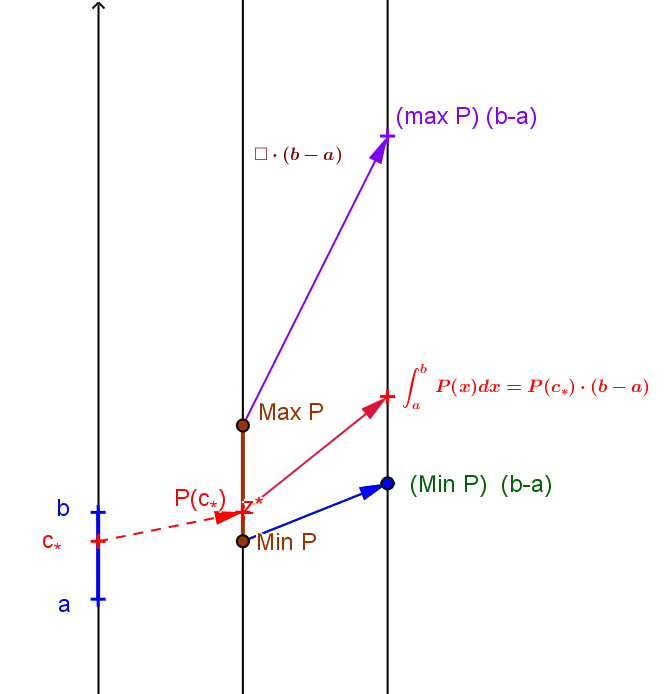CIS.VMVTI. Visualization of the Mean Value Theorem for Integrals
Theorem: Suppose $P$ is a continuous function on $[a,b]$. Then for some $c\in (a,b)$,
$P(c)[b−a]=\int_a^b P(x)dx$ or $P(c)=\frac{\int_a^b P(x)dx}{b−a}$.

Comment: The mean value theorem for integrals and its proof are usually visualized using the area interpretation of the definite integral, connecting the definite integral with measuring "area" of regions in the plane. Using mapping diagrams in the visualization of this result connects the definite integral with the accumulation of change.
Interpretations:
 Suppose $P$ is a continuous function on $[a,b]$. Then for some $c\in (a,b)$, $P(c)[b−a]=\int_a^b P(x)dx$ is interpreted as: the area of the region in the plane enclosed by the segment $[a,b]$ on the X-axis, the lines $x=a$ and $x=b$, and the graph of $y=P(x)$ is equal to the area of the rectangle in the plane with base the segment $[a,b]$ on the X-axis and "height" $P(c)$. Suppose $P$ is a continuous function on $[a,b]$.  Then for some $c\in (a,b)$, $P(c)[b−a]=\int_a^b P(x)dx$ is interpreted as: the measure of the change for a quantity $y$ during the interval $[a,b]$ , when  $\frac{dy}{dx}=P(x)$ is equal to the change for a quantity for the same interval $[a,b]$,when  $\frac{dy}{dx}=P(c_*)$.